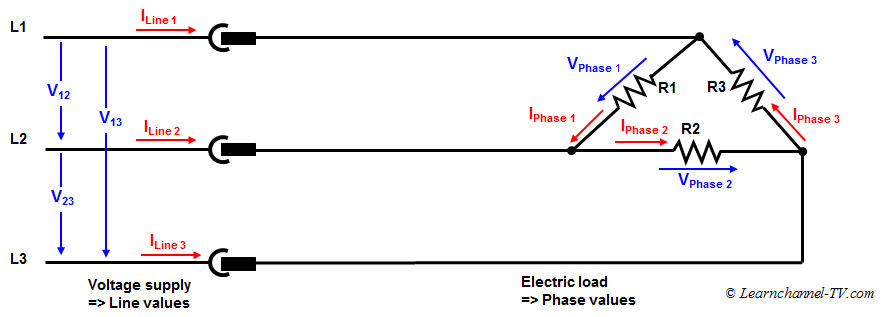## Delta Connection

Delta Connection is obtained when the opposite ends of the three resisors are connected together. Or in other words: The end of each resistor are connected with the starting point of another resistor and the three wires are taken out from the resistor joints.3 Phase Current - Delta-connection - Voltage and Current values

What you can see from this circuit: In Delta connection the Line Voltage is Equal to the Phase Voltage:

VLine = VPhase

A line current branches out into two phase currents.

ILine  =  √3  IPhase